Next: Beam Field Up: Physics and Numerical Methods Previous: Equation of motion under

## Luminosity Integration

Let us denote the position-velocity distribution function of j-th beam (j=1,2) at time t by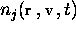. It is normalized such that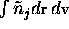is the total number of particles in the j-th beam. The luminosity (per crossing) is in general given by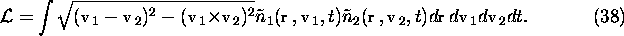If all the particles in the j-th beam are ultrarelativistic and have almost the same velocity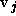(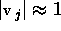), then the expression is simplified as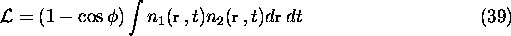where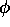is the polar angle between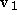and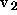, and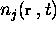is the number density of the j-th beam. CAIN uses this formula with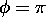, ignoring the velocity distribution and the crossing angle.

The integration is done by introducing the time step size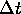, longitudinal slice width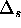, transverse mesh size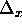and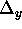. Summing the number of particles in each bin, the luminosity is given by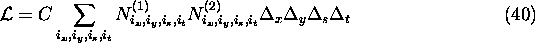where C is an appropriate normalization factor, and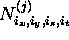is the number of particles of the beam j in the bin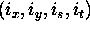. A problem is how to determine the transverse size  of the bin (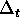andis mainly determined by the dynamics -- they are actually specified by the user). If the bin is too large, detail of the distribution is lost, whereas if too small, statistical error becomes large because each bin will contain only a small number of macro-particles. CAIN adopts the following way.

At first, determine the size of the whole transverse region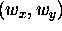such that most particles are contained there. Then, divide this region into as many bins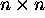as allowed by the storage requirement (n must be a power of 2. CAIN uses n=128.), and count the number of particles in each bin for both beams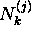(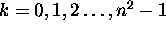).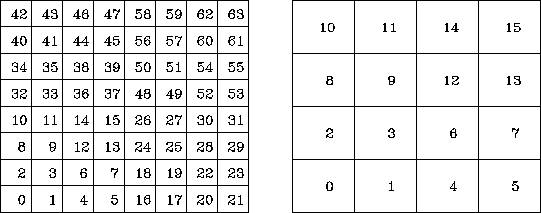Figure 2: Bin numbering for luminosity integration. Example with n=8 and the double-sized bin n=4.

If the number of macro-particles in any of the neighbouring 4 bins are less than some number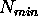(CAIN adopts 5) for both beams, then sum these numbers and put the sum into a larger bin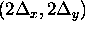. (For the example in Fig.2, the sum of the bins 12, 13, 14, and 15 in the figure on the left corresponds to the bin 3 on the right.) Otherwise, add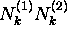into the luminosity sum. This doubling of the bin size is repeated so long as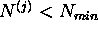. In order to make this algorithm efficient, the bin numbering system is a little complicated. Instead of using two indices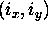, the bins are numbered as in Fig.2. With this numbering, the sum of neighbouring bins can be simply written as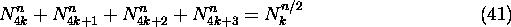where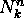is the number of particles in the k-th bin (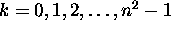) inbin system.Next: Beam Field Up: Physics and Numerical Methods Previous: Equation of motion under

Toshiaki Tauchi
Thu Dec 3 17:27:26 JST 1998